# RRB ALP 2018 Practice Test Papers | Reasoning Questions (Day-51)

Dear Aspirants, Here we have given the Important RRB ALP & Technicians Exam 2018 Practice Test Papers. Candidates those who are preparing for RRB ALP 2018 can practice these Reasoning Questions to get more confidence to Crack RRB 2018 Examination.

[WpProQuiz 2656]

Click “Start Quiz” to attend these Questions and view Solutions

1. From the given answer figures, select the one in which the question figure is hidden/embedded.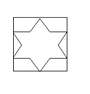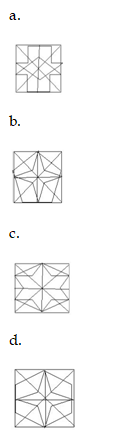1. Which one of the given response would be a meaningful ascending order of the following?

1.India

2.Universe

3.Planet

4.Asia

5.World

1. 14523
2. 24351
3. 23451
4. 45123
1. Pointing to a girl, Suresh said “She is the Daughter of my grandfather’s only son”. How is the Girl related to Suresh?
1. Cousin
2. Niece
3. Sister
4. Aunt
1. Rohan walked 10 km to the West, then turned right and walked10 km, then turned right and walked 5 km and then turned right again and walked 5 km and then turned left and walked 5 km. How far is he from the starting point?
1. 7km
2. 10km
3. 15 km
4. 5km
1. Find the Missing Numbers

2, 1.5, 2.5, 7, 32, ?

1. 256
2. 264
3. 278
4. 245

6.There are 5 boys of different age groups. Gokul is older than shah, but younger than Mirsha. Shah is second youngest boy in the group. Hati is not youngest boy in the group and is elder than Mirsha. Who is the second oldest boy in the group?

1. Mirsha
2. Hati
3. Gokul
4. Shah
1. If 4×6×1 = 101

2×8×2 = 70

then 6× 1×3 = ?

1. 60
2. 50
3. 40
4. 20
1. Select the related letters

CDXW: GHTS::KLPO: ?

1. OPLK
2. OKLP
3. FGTU
4. QRDE
1. Statement

All notes are book

All books are pen

All pencils are pen

Conclusion

Some books are pen

Some Pencils are Notes

1. Only I follow
2. Only II Follow
3. Both Follows
4. None Follows
1. Statement

K=PO<RS=T<MA

I.R > M

II.PS

1. Only I follow
2. Only II follow
3. Either I or II follow
4. Neither I nor II follow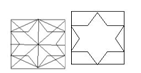Universe > Planet > World > Asia > India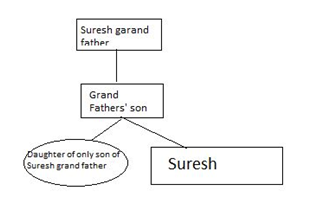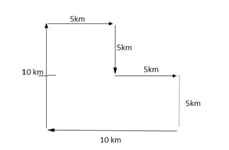2×0.5 + 0.5 = 1.5

1.5 × 1+ 1= 2.5

2.5 × 2 + 2 =7

7 × 4 + 4 = 32

32× 8 + 8 = 264

Hati>Mirsha>Gokul>Shah> ________(unnamed)

4×6×1 = 101 = 42 + 62 + 1 = 101

2×8×2 = 70 = 22 + 82+2 = 70

6× 1×3= ? = 62 + 12 + 3 = 36+1+3 =40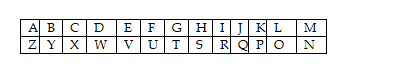CDXW: GHTS

CD coded as reverse Alphabetic order XW

C+3 = G

GH coded as reverse Alphabetic order TS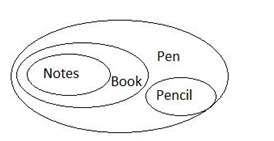K=P≤O<R≥S=T<M≤A

I.R > M does not follow

II.P≤S Does not follow

RRB ALP 2018 – “All in One” Study Materials and Practice Sets